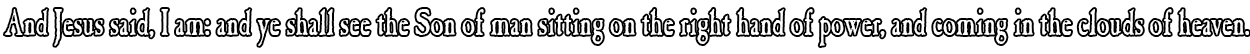None:
Polyps:
Strongs:Mathematics Introduction The proof that A5 (and all An for n>5) is a non-abelian simple group is not given in the book. The book simply asks the reader to tacitly accept this fact. The proof, given in stages over several pages and drawn together at the end of the section was supplied to me as an undergraduate by the lecturer for my 'Group Theory' course. Whilst not the only proof that A5 is simple, it is easy enough a proof for me to understand and so I present the same argument here. Simple Groups This page states the basic properties of Abelian groups (those groups with a commutative operation), which always factor into simple groups: a series of prime order cyclic groups. (I state the fundamental theorem of finitely generated abelian groups.) Also stated is the relationship between the order of Sn and An, the symmetric group of degree n and its largest non-trivial normal subgroup, the alternating group of degree n. An Is Generated By 3-Cycles A simple argument to show that every alternating group of degree n>3 is generated by products of 3-cycles. (3-cycles are themselves a product of an even number of transpositions.) Every element of An (n>3) is a product of 3-cycles in its 'supergroup' Sn. Every Normal Subgroup of An contains Every 3-Cycle in An Another simple argument; this time to show that if H is a normal subgroup of An, (which includes An itself) then it is true that every 3-cycle in An is also in H. Every Normal Subgroup Of An Contains A 3-Cycle A somewhat lengthier argument to show that every normal subgroup H of An contains at least one 3-cycle from Sn is presented here. I state that this proof is the hardest of the proofs in this section to remember, but is reasonably simple once you understand how the products of permutations operate in their cycle notation. An (n>4) Is A Simple Group In drawing together all the threads from this section it is almost a simple observation that An for all n>4 are simple groups. (Of course, A5 is simple - as is every alternating group of higher degree.) Continue To Next Section Continue To Next Page'